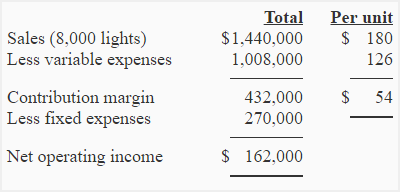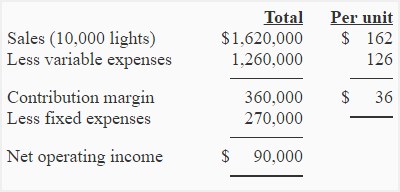# Exercise-1 (Target profit analysis, break-even point)

PNG electric company manufactures a number of electric products. Rechargeable light is one of the PNG’s products that sells for \$180/unit. Total fixed expenses related to rechargeable electric light are \$270,000 per month and variable expenses involved in manufacturing this product are \$126 per unit. Monthly sales are 8,000 rechargeable lights.

Required:

1. Compute break-even point of the company in dollars and units.
2. According to a research conducted by sales department, a 10% reduction in sales price will result in 25% increase in unit sale. Prepare two income statements in contribution margin format, one using the current price and one using proposed price (10% below the old sales price).
3. Compute the number of rechargeable lights to be sold to earn a net operating income of \$189,000 per month (use original data).

## Solution:

#### (1) Computation of break-even point:

a. Break even point in units:

Break even point in units can be computed by using either equation method or contribution margin method. Both the methods are given below:

Equation method:

Sales = Variable expenses + Fixed expenses

\$180Q = \$126Q + 270,000

\$180Q – \$126Q = \$270,000

\$54Q = \$270,000

Q = \$270,000/\$54

Q = 5,000 Units

Contribution margin method:

Break even point = Fixed expenses/Contribution margin per unit

270,000 / 54*

= 5,000 units

*\$180 – \$126

b. Break-even point in dollars:

Break-even point in dollars can be computed by multiplying break-even point in units by sales price per unit as shown below:

5,000 units × \$180

=\$900,000

#### (2) Income statements:

a. Income statement under current operations:b. Income statement under proposed operations:The proposal should not be accepted because it will reduce the contribution margin from \$54 per unit to \$36 per unit and net operating income from \$162,000 to \$90,000.

#### (3) Target profit analysis:

a. Equation method:

Sales = Variable expenses + Fixed expenses + Profit

\$180Q = \$126Q + 270,000 + \$189,000

\$180Q – \$126Q = \$459,000

\$54Q = \$459,000

Q = \$459,000 / \$54

Q = 8,500 Units

b. Contribution margin method:

(Fixed expenses + Target income)/Contribution margin per unit

= (\$270,000+\$189,000)/54

= 8,500 units

On the basis of original data, company needs to sell 8,500 rechargeable lights to earn a profit of \$189,000.

A D V E R T I S E M E N T
14 Comments on Exercise-1 (Target profit analysis, break-even point)
1.Kassahun

Yes,I am very much helped in your detail explanation.

2.Alexa

Third question: “Compute the number of rechargeable lights to be sold to earn a net operating income of \$144,000 per month.” Answer given for 72.000, should be 11500 units.

3.zaid

3ed quastion i think the answ0er shuld be like this 144000+270000/cm(54)=7666 units

1.Accounting For Management

\$270000+\$189000/cm(54)=8500 units

4.Razaz Eliass

can i have more explaine about the target profit analysis

1.Accounting For Management
5.Chris

she doen’t like me to control her life as well as i can’t control of the sale arise or goes down. so learning this formula make realize that how to make balance between love and sale.

6.Lucky

Sale of 8,000 units @ 8
Prime cost 3 Rs.
Fixed cost 1,00,000 Rs.
Calculate BEP and MOP..??

1.100000/8-8=20000ans

7.ale

Income statement under proposed operations:

Can anyone explain how did we get 10,000 unit price and 162 per unit?

1.Accounting For Management

Under proposed operations, we assume that the sale price will reduce by 10% and the number of units sold will increase by 25%.

Number of units sold under proposed operations:
8,000 + (8,000 x 25%) = 10,000 units

Sale price under proposed operations:
180 – (180 x 10%) = \$162

8.mengistu

thanks

9.Amere Moges

10.